# Weight watcher Bay Area ACM Feb 28, 2022

Feb. 28, 2022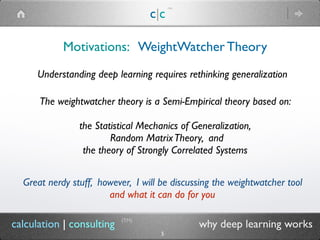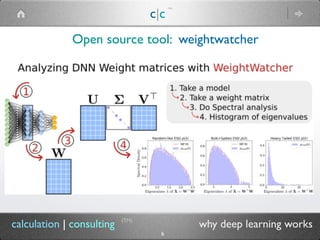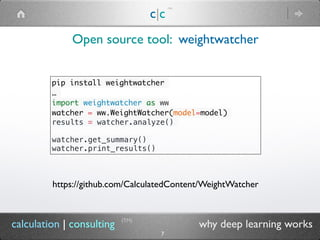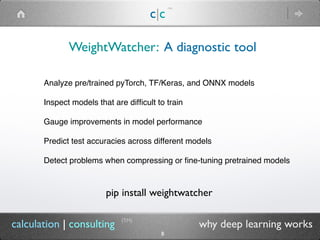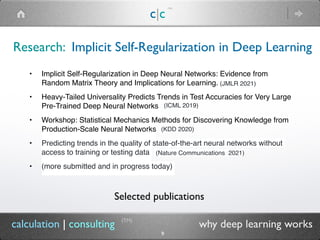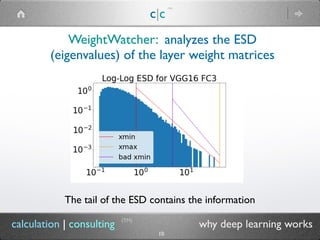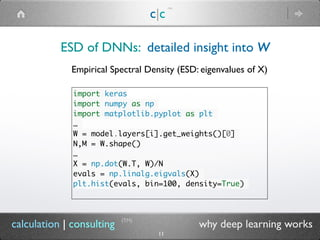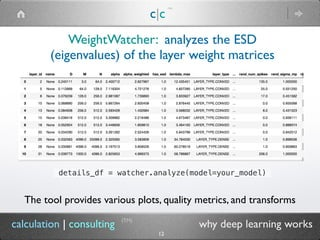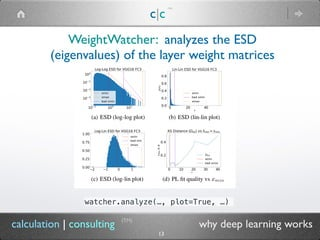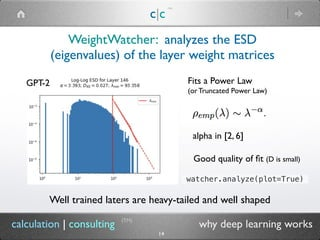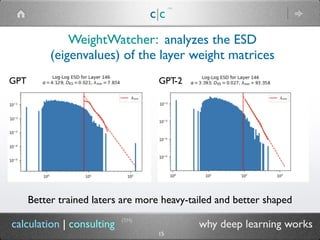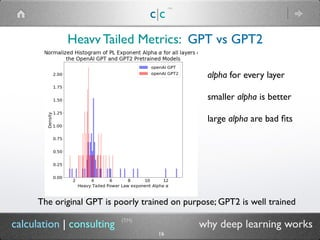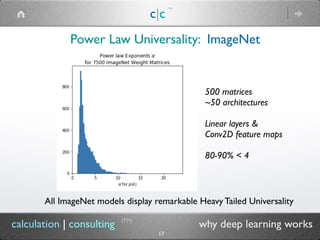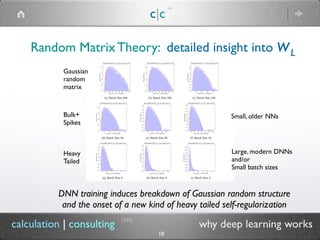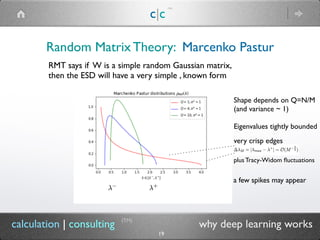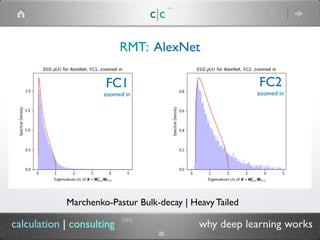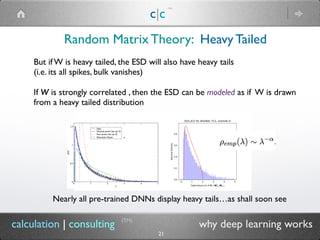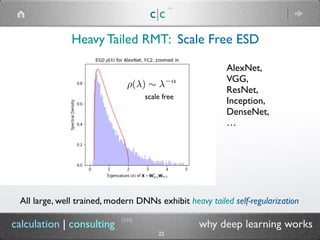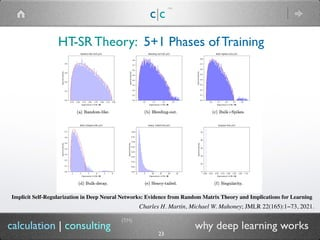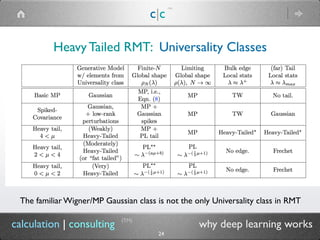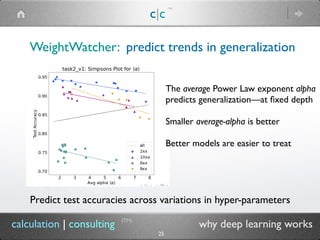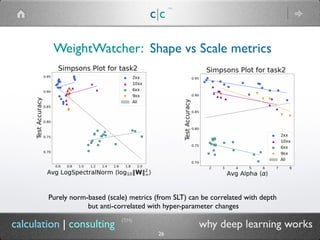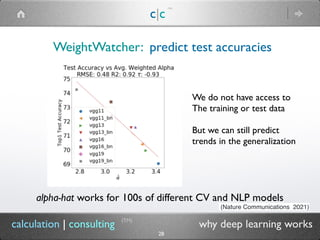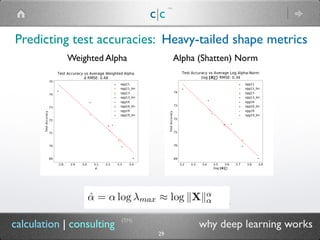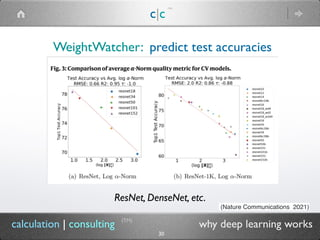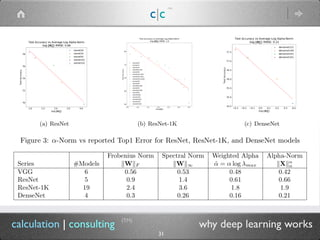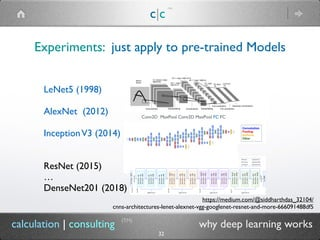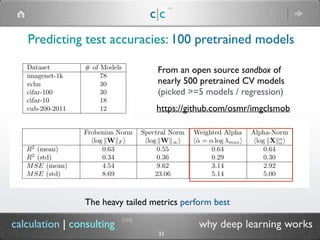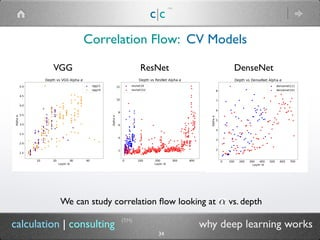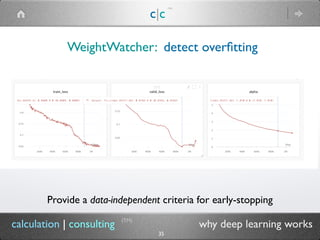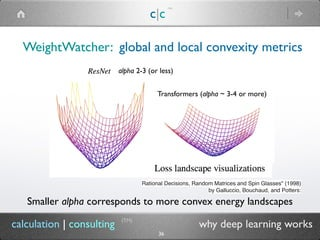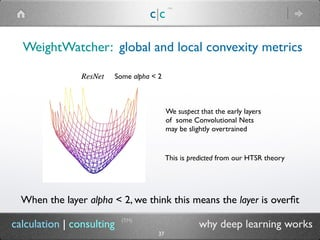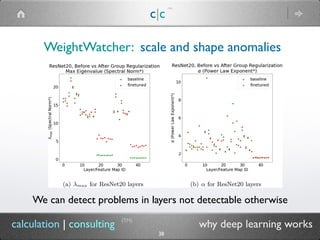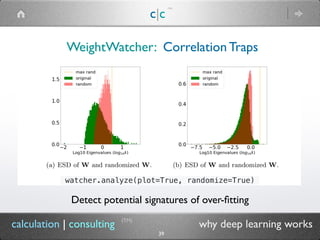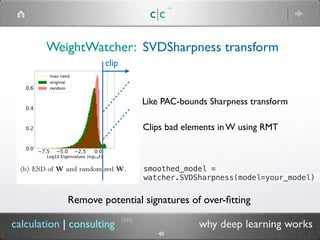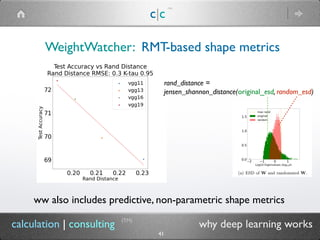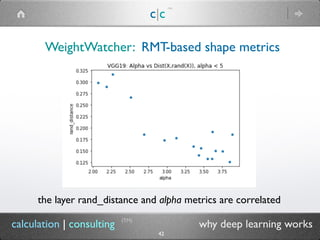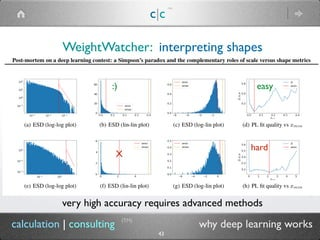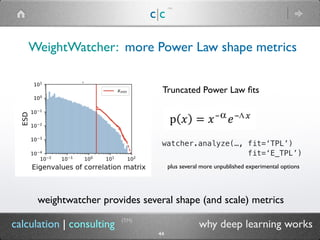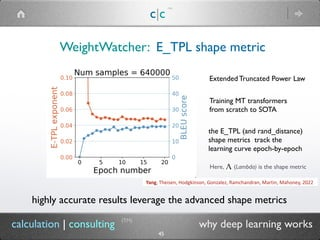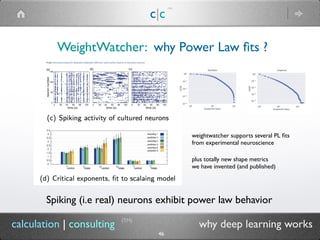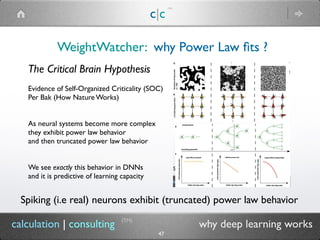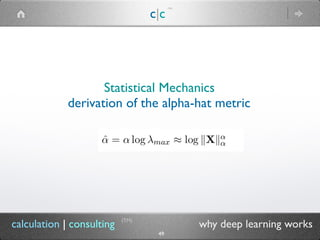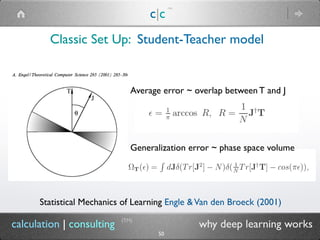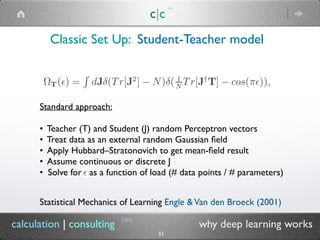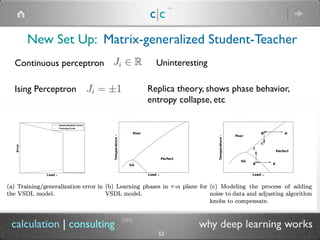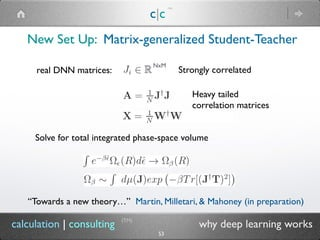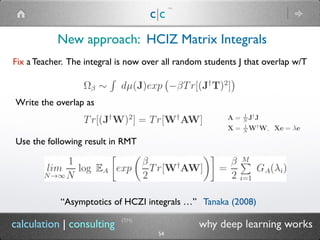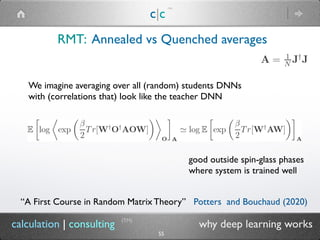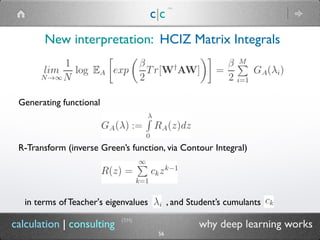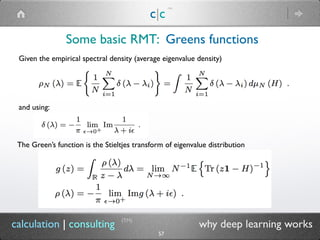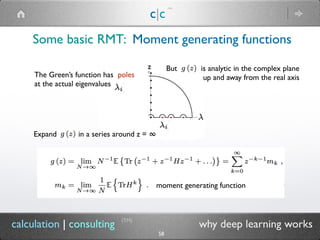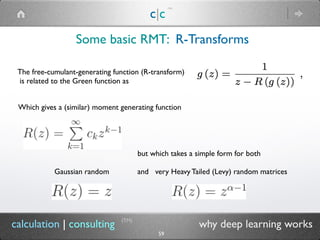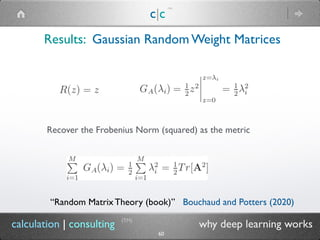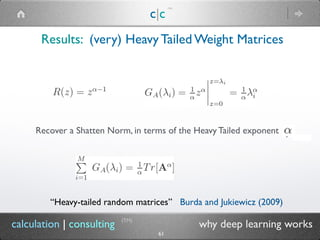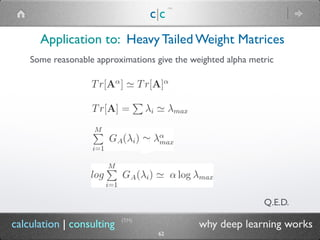1 of 63

### Weight watcher Bay Area ACM Feb 28, 2022

• 1. calculation | consulting weightwatcher - a diagnostic tool for deep neural networks (TM) c|c (TM) charles@calculationconsulting.com
• 2. calculation|consulting weightwatcher - a diagnostic tool for deep neural networks (TM) charles@calculationconsulting.com
• 3. calculation | consulting why deep learning works Who Are We? c|c (TM) Dr. Charles H. Martin, PhD University of Chicago, Chemical Physics NSF Fellow in Theoretical Chemistry, UIUC Over 15 years experience in applied Machine Learning and AI ML algos for: Aardvark, acquired by Google (2010) Demand Media (eHow); first \$1B IPO since Google Wall Street: BlackRock Fortune 500: Roche, France Telecom BigTech: eBay, Aardvark (Google), GoDaddy Private Equity: Griffin Advisors Alt. Energy: Anthropocene Institute (Page Family) www.calculationconsulting.com charles@calculationconsulting.com (TM) 3
• 4. calculation | consulting why deep learning works c|c (TM) (TM) 4 Michael W. Mahoney ICSI, RISELab, Dept. of Statistics UC Berkeley Algorithmic and statistical aspects of modern large-scale data analysis. large-scale machine learning | randomized linear algebra geometric network analysis | scalable implicit regularization PhD, Yale University, computational chemical physics SAMSI National Advisory Committee NRC Committee on the Analysis of Massive Data Simons Institute Fall 2013 and 2018 program on the Foundations of Data Biennial MMDS Workshops on Algorithms for Modern Massive Data Sets NSF/TRIPODS-funded Foundations of Data Analysis Institute at UC Berkeley https://www.stat.berkeley.edu/~mmahoney/ mmahoney@stat.berkeley.edu Who Are We?
• 5. c|c (TM) (TM) 5 calculation | consulting why deep learning works Understanding deep learning requires rethinking generalization Motivations: WeightWatcher Theory The weightwatcher theory is a Semi-Empirical theory based on:  the Statistical Mechanics of Generalization, Random MatrixTheory, and the theory of Strongly Correlated Systems Great nerdy stuff, however, I will be discussing the weightwatcher tool and what it can do for you
• 6. c|c (TM) (TM) 6 calculation | consulting why deep learning works Open source tool: weightwatcher
• 7. c|c (TM) (TM) 7 calculation | consulting why deep learning works Open source tool: weightwatcher pip install weightwatcher … import weightwatcher as ww watcher = ww.WeightWatcher(model=model) results = watcher.analyze() watcher.get_summary() watcher.print_results() https://github.com/CalculatedContent/WeightWatcher
• 8. c|c (TM) WeightWatcher: A diagnostic tool (TM) 8 calculation | consulting why deep learning works Analyze pre/trained pyTorch, TF/Keras, and ONNX models Inspect models that are difficult to train Gauge improvements in model performance Predict test accuracies across different models Detect problems when compressing or fine-tuning pretrained models pip install weightwatcher
• 9. c|c (TM) Research: Implicit Self-Regularization in Deep Learning (TM) 9 calculation | consulting why deep learning works • Implicit Self-Regularization in Deep Neural Networks: Evidence from Random Matrix Theory and Implications for Learning. • Heavy-Tailed Universality Predicts Trends in Test Accuracies for Very Large Pre-Trained Deep Neural Networks • Workshop: Statistical Mechanics Methods for Discovering Knowledge from Production-Scale Neural Networks • Predicting trends in the quality of state-of-the-art neural networks without access to training or testing data • (more submitted and in progress today) (JMLR 2021) (ICML 2019) (KDD 2020) (Nature Communications 2021) Selected publications
• 10. c|c (TM) WeightWatcher: analyzes the ESD (eigenvalues) of the layer weight matrices (TM) 10 calculation | consulting why deep learning works The tail of the ESD contains the information
• 11. c|c (TM) (TM) 11 calculation | consulting why deep learning works ESD of DNNs: detailed insight into W Empirical Spectral Density (ESD: eigenvalues of X) import keras import numpy as np import matplotlib.pyplot as plt … W = model.layers[i].get_weights() N,M = W.shape() … X = np.dot(W.T, W)/N evals = np.linalg.eigvals(X) plt.hist(evals, bin=100, density=True)
• 12. c|c (TM) WeightWatcher: analyzes the ESD (eigenvalues) of the layer weight matrices (TM) 12 calculation | consulting why deep learning works details_df = watcher.analyze(model=your_model) The tool provides various plots, quality metrics, and transforms
• 13. c|c (TM) WeightWatcher: analyzes the ESD (eigenvalues) of the layer weight matrices (TM) 13 calculation | consulting why deep learning works watcher.analyze(…, plot=True, …)
• 14. c|c (TM) WeightWatcher: analyzes the ESD (eigenvalues) of the layer weight matrices (TM) 14 calculation | consulting why deep learning works Well trained laters are heavy-tailed and well shaped GPT-2 Fits a Power Law (or Truncated Power Law) alpha in [2, 6] watcher.analyze(plot=True) Good quality of fit (D is small)
• 15. c|c (TM) WeightWatcher: analyzes the ESD (eigenvalues) of the layer weight matrices (TM) 15 calculation | consulting why deep learning works Better trained laters are more heavy-tailed and better shaped GPT GPT-2
• 16. c|c (TM) (TM) 16 calculation | consulting why deep learning works Heavy Tailed Metrics: GPT vs GPT2 The original GPT is poorly trained on purpose; GPT2 is well trained alpha for every layer  smaller alpha is better large alpha are bad fits
• 17. c|c (TM) (TM) 17 calculation | consulting why deep learning works Power Law Universality: ImageNet All ImageNet models display remarkable Heavy Tailed Universality 500 matrices ~50 architectures Linear layers & Conv2D feature maps 80-90% < 4
• 18. c|c (TM) (TM) 18 calculation | consulting why deep learning works Random Matrix Theory: detailed insight into WL DNN training induces breakdown of Gaussian random structure and the onset of a new kind of heavy tailed self-regularization Gaussian random matrix Bulk+ Spikes Heavy Tailed Small, older NNs Large, modern DNNs and/or Small batch sizes
• 19. c|c (TM) (TM) 19 calculation | consulting why deep learning works Random Matrix Theory: Marcenko Pastur plus Tracy-Widom fluctuations very crisp edges Q RMT says if W is a simple random Gaussian matrix, then the ESD will have a very simple , known form Shape depends on Q=N/M (and variance ~ 1) Eigenvalues tightly bounded a few spikes may appear
• 20. c|c (TM) (TM) 20 calculation | consulting why deep learning works RMT: AlexNet Marchenko-Pastur Bulk-decay | Heavy Tailed FC1 zoomed in FC2 zoomed in
• 21. c|c (TM) (TM) 21 calculation | consulting why deep learning works Random Matrix Theory: Heavy Tailed But if W is heavy tailed, the ESD will also have heavy tails (i.e. its all spikes, bulk vanishes) If W is strongly correlated , then the ESD can be modeled as if W is drawn from a heavy tailed distribution Nearly all pre-trained DNNs display heavy tails…as shall soon see
• 22. c|c (TM) (TM) 22 calculation | consulting why deep learning works AlexNet,   VGG, ResNet, Inception, DenseNet, … Heavy Tailed RMT: Scale Free ESD All large, well trained, modern DNNs exhibit heavy tailed self-regularization scale free
• 23. c|c (TM) (TM) 23 calculation | consulting why deep learning works HT-SR Theory: 5+1 Phases of Training Implicit Self-Regularization in Deep Neural Networks: Evidence from Random Matrix Theory and Implications for Learning Charles H. Martin, Michael W. Mahoney; JMLR 22(165):1−73, 2021.
• 24. c|c (TM) (TM) 24 calculation | consulting why deep learning works Heavy Tailed RMT: Universality Classes The familiar Wigner/MP Gaussian class is not the only Universality class in RMT
• 25. c|c (TM) WeightWatcher: predict trends in generalization (TM) 25 calculation | consulting why deep learning works Predict test accuracies across variations in hyper-parameters The average Power Law exponent alpha predicts generalization—at fixed depth   Smaller average-alpha is better Better models are easier to treat
• 26. c|c (TM) WeightWatcher: Shape vs Scale metrics (TM) 26 calculation | consulting why deep learning works Purely norm-based (scale) metrics (from SLT) can be correlated with depth but anti-correlated with hyper-parameter changes
• 27. c|c (TM) WeightWatcher: treat architecture changes (TM) 27 calculation | consulting why deep learning works Predict test accuracies across variations in hyper-parameters and depth The alpha-hat metric combines shape and scale metrics and corrects for different depths (grey line) can be derived from theory…
• 28. c|c (TM) WeightWatcher: predict test accuracies (TM) 28 calculation | consulting why deep learning works alpha-hat works for 100s of different CV and NLP models (Nature Communications 2021) We do not have access to The training or test data But we can still predict trends in the generalization
• 29. c|c (TM) (TM) 29 calculation | consulting why deep learning works Predicting test accuracies: Heavy-tailed shape metrics The heavy tailed metrics perform best Weighted Alpha Alpha (Shatten) Norm
• 30. c|c (TM) WeightWatcher: predict test accuracies (TM) 30 calculation | consulting why deep learning works ResNet, DenseNet, etc. (Nature Communications 2021)
• 31. c|c (TM) (TM) 31 calculation | consulting why deep learning works
• 32. c|c (TM) (TM) 32 calculation | consulting why deep learning works Experiments: just apply to pre-trained Models LeNet5 (1998) AlexNet (2012) InceptionV3 (2014) ResNet (2015) … DenseNet201 (2018) https://medium.com/@siddharthdas_32104/ cnns-architectures-lenet-alexnet-vgg-googlenet-resnet-and-more-666091488df5 Conv2D MaxPool Conv2D MaxPool FC FC
• 33. c|c (TM) (TM) 33 calculation | consulting why deep learning works Predicting test accuracies: 100 pretrained models The heavy tailed metrics perform best https://github.com/osmr/imgclsmob From an open source sandbox of nearly 500 pretrained CV models (picked >=5 models / regression)
• 34. c|c (TM) (TM) 34 calculation | consulting why deep learning works Correlation Flow: CV Models We can study correlation flow looking at vs. depth VGG ResNet DenseNet
• 35. c|c (TM) WeightWatcher: detect overfitting (TM) 35 calculation | consulting why deep learning works Provide a data-independent criteria for early-stopping
• 36. c|c (TM) WeightWatcher: global and local convexity metrics (TM) 36 calculation | consulting why deep learning works Smaller alpha corresponds to more convex energy landscapes Transformers (alpha ~ 3-4 or more) alpha 2-3 (or less) Rational Decisions, Random Matrices and Spin Glasses" (1998) by Galluccio, Bouchaud, and Potters:
• 37. c|c (TM) WeightWatcher: global and local convexity metrics (TM) 37 calculation | consulting why deep learning works When the layer alpha < 2, we think this means the layer is overfit We suspect that the early layers of some Convolutional Nets may be slightly overtrained Some alpha < 2 This is predicted from our HTSR theory
• 38. c|c (TM) WeightWatcher: scale and shape anomalies (TM) 38 calculation | consulting why deep learning works We can detect problems in layers not detectable otherwise
• 39. c|c (TM) (TM) 39 calculation | consulting why deep learning works Detect potential signatures of over-fitting WeightWatcher: Correlation Traps watcher.analyze(plot=True, randomize=True)
• 40. c|c (TM) WeightWatcher: SVDSharpness transform (TM) 40 calculation | consulting why deep learning works Remove potential signatures of over-fitting Like PAC-bounds Sharpness transform Clips bad elements in W using RMT clip smoothed_model = watcher.SVDSharpness(model=your_model)
• 41. c|c (TM) WeightWatcher: RMT-based shape metrics (TM) 41 calculation | consulting why deep learning works ww also includes predictive, non-parametric shape metrics rand_distance = jensen_shannon_distance(original_esd, random_esd)
• 42. c|c (TM) WeightWatcher: RMT-based shape metrics (TM) 42 calculation | consulting why deep learning works the layer rand_distance and alpha metrics are correlated
• 43. c|c (TM) WeightWatcher: interpreting shapes (TM) 43 calculation | consulting why deep learning works very high accuracy requires advanced methods hard easy X :)
• 44. c|c (TM) WeightWatcher: more Power Law shape metrics (TM) 44 calculation | consulting why deep learning works watcher.analyze(…, fit=‘TPL’) Truncated Power Law fits fit=‘E_TPL’) weightwatcher provides several shape (and scale) metrics plus several more unpublished experimental options
• 45. c|c (TM) WeightWatcher: E_TPL shape metric (TM) 45 calculation | consulting why deep learning works the E_TPL (and rand_distance) shape metrics track the learning curve epoch-by-epoch Training MT transformers from scratch to SOTA Extended Truncated Power Law highly accurate results leverage the advanced shape metrics Here, (Lambda) is the shape metric
• 46. c|c (TM) WeightWatcher: why Power Law fits ? (TM) 46 calculation | consulting why deep learning works Spiking (i.e real) neurons exhibit power law behavior weightwatcher supports several PL fits from experimental neuroscience plus totally new shape metrics we have invented (and published)
• 47. c|c (TM) WeightWatcher: why Power Law fits ? (TM) 47 calculation | consulting why deep learning works Spiking (i.e real) neurons exhibit (truncated) power law behavior The Critical Brain Hypothesis Evidence of Self-Organized Criticality (SOC) Per Bak (How Nature Works) As neural systems become more complex they exhibit power law behavior and then truncated power law behavior We see exactly this behavior in DNNs and it is predictive of learning capacity
• 48. c|c (TM) WeightWatcher: open-source, open-science (TM) 48 calculation | consulting why deep learning works We are looking for early adopters and collaborators github.com/CalculatedContent/WeightWatcher We have a Slack channel to support the tool Please file issues Ping me to join
• 49. c|c (TM) (TM) 49 calculation | consulting why deep learning works Statistical Mechanics derivation of the alpha-hat metric
• 50. c|c (TM) (TM) 50 calculation | consulting why deep learning works Classic Set Up: Student-Teacher model Statistical Mechanics of Learning Engle &Van den Broeck (2001) Generalization error ~ phase space volume Average error ~ overlap between T and J
• 51. c|c (TM) (TM) 51 calculation | consulting why deep learning works Classic Set Up: Student-Teacher model Statistical Mechanics of Learning Engle &Van den Broeck (2001) Standard approach: • Teacher (T) and Student (J) random Perceptron vectors • Treat data as an external random Gaussian field • Apply Hubbard–Stratonovich to get mean-field result • Assume continuous or discrete J • Solve for as a function of load (# data points / # parameters)
• 52. c|c (TM) (TM) 52 calculation | consulting why deep learning works New Set Up: Matrix-generalized Student-Teacher x Continuous perceptron Ising Perceptron Uninteresting Replica theory, shows phase behavior, entropy collapse, etc
• 53. c|c (TM) (TM) 53 calculation | consulting why deep learning works New Set Up: Matrix-generalized Student-Teacher “Towards a new theory…” Martin, Milletari, & Mahoney (in preparation) real DNN matrices: NxM Strongly correlated Heavy tailed correlation matrices Solve for total integrated phase-space volume
• 54. c|c (TM) (TM) 54 calculation | consulting why deep learning works New approach: HCIZ Matrix Integrals Write the overlap as Fix a Teacher. The integral is now over all random students J that overlap w/T Use the following result in RMT “Asymptotics of HCZI integrals …” Tanaka (2008)
• 55. c|c (TM) (TM) 55 calculation | consulting why deep learning works RMT: Annealed vs Quenched averages “A First Course in Random Matrix Theory” Potters and Bouchaud (2020) good outside spin-glass phases where system is trained well We imagine averaging over all (random) students DNNs with (correlations that) look like the teacher DNN
• 56. c|c (TM) (TM) 56 calculation | consulting why deep learning works New interpretation: HCIZ Matrix Integrals Generating functional R-Transform (inverse Green’s function, via Contour Integral) in terms of Teacher's eigenvalues , and Student’s cumulants
• 57. c|c (TM) (TM) 57 calculation | consulting why deep learning works Some basic RMT: Greens functions The Green’s function is the Stieltjes transform of eigenvalue distribution Given the empirical spectral density (average eigenvalue density) and using:
• 58. c|c (TM) (TM) 58 calculation | consulting why deep learning works Some basic RMT: Moment generating functions The Green’s function has poles at the actual eigenvalues But is analytic in the complex plane up and away from the real axis z Expand in a series around z = ∞ moment generating function
• 59. c|c (TM) (TM) 59 calculation | consulting why deep learning works Some basic RMT: R-Transforms Which gives a (similar) moment generating function The free-cumulant-generating function (R-transform) is related to the Green function as Gaussian random and very Heavy Tailed (Levy) random matrices but which takes a simple form for both
• 60. c|c (TM) (TM) 60 calculation | consulting why deep learning works Results: Gaussian Random Weight Matrices “Random Matrix Theory (book)” Bouchaud and Potters (2020) Recover the Frobenius Norm (squared) as the metric
• 61. c|c (TM) (TM) 61 calculation | consulting why deep learning works Results: (very) Heavy Tailed Weight Matrices “Heavy-tailed random matrices” Burda and Jukiewicz (2009) Recover a Shatten Norm, in terms of the Heavy Tailed exponent
• 62. c|c (TM) (TM) 62 calculation | consulting why deep learning works Application to: Heavy Tailed Weight Matrices Some reasonable approximations give the weighted alpha metric Q.E.D.
• 63. (TM) c|c (TM) c | c charles@calculationconsulting.com